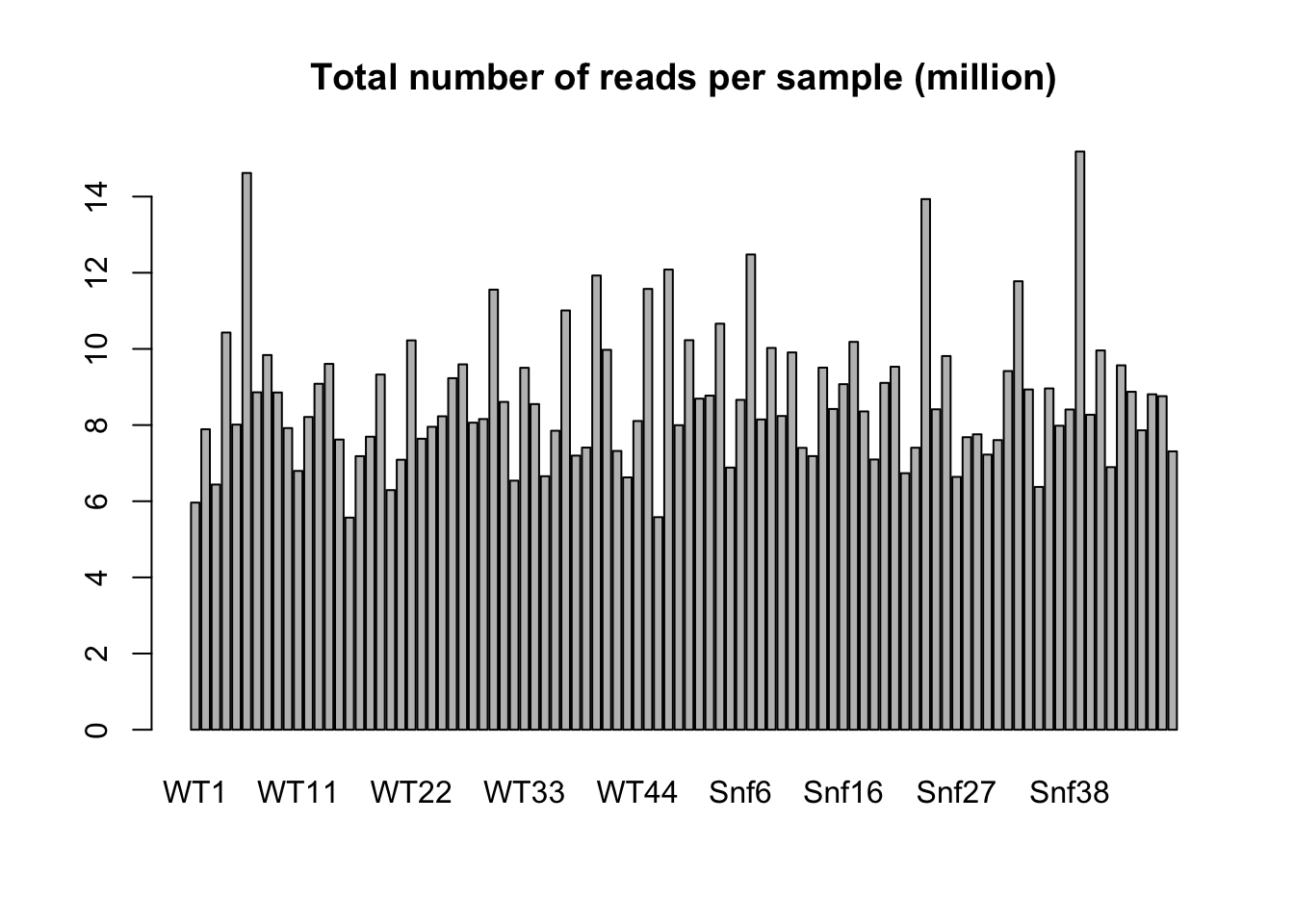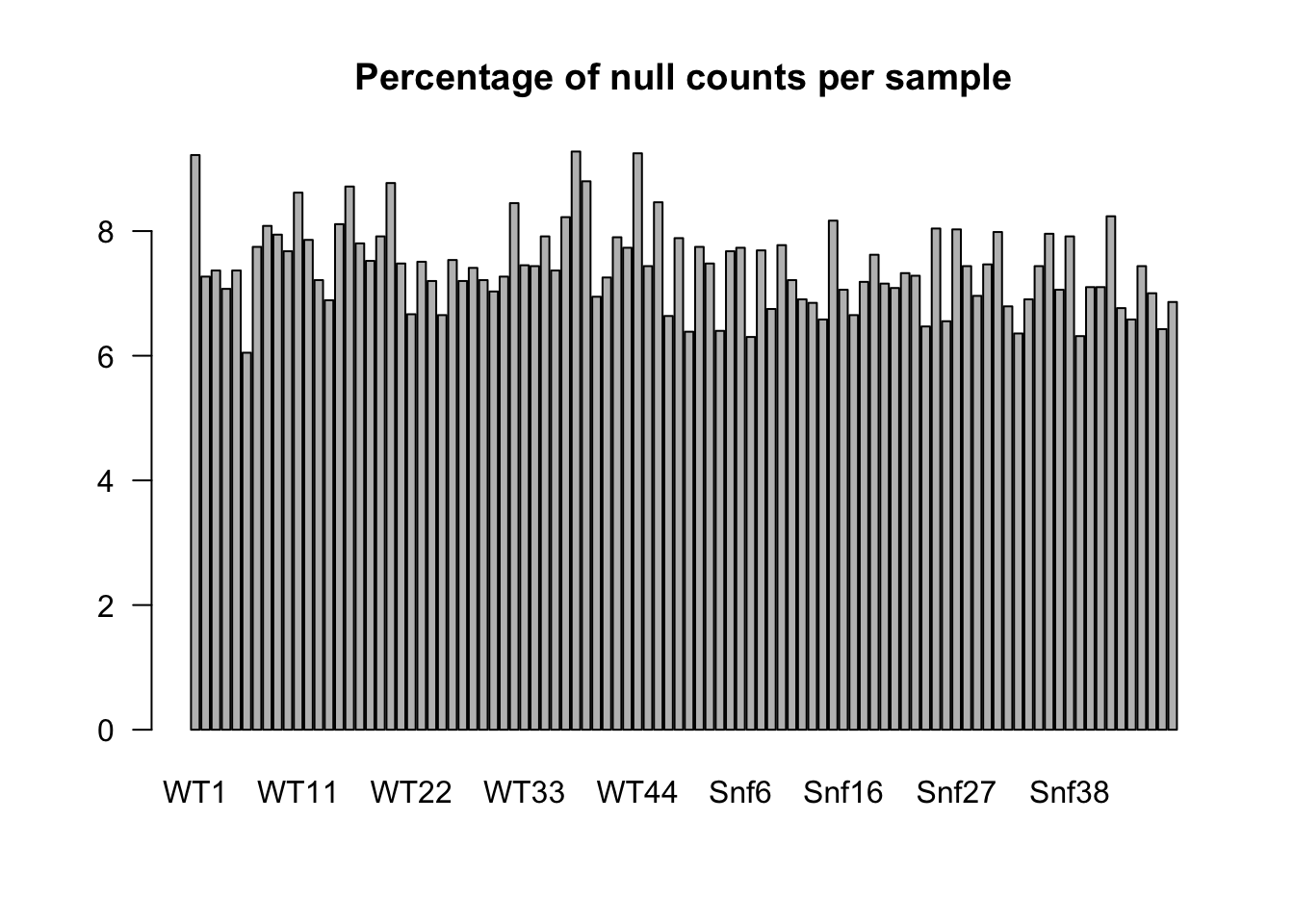2017-01-08

# Requirements

For people using their own laptop, install some R packages:

``````source("http://bioconductor.org/biocLite.R")
if (!require(DESeq2)) {
}
if (!require(edgeR)) {
}
if (!require(gplots)) {
}``````

# Context

• Study of 48 WT yeast samples vs 48 Snf2 (KO) samples:

1. Gierliński,M., Cole,C., Schofield,P., Schurch,N.J., Sherstnev,A., Singh,V., Wrobel,N., Gharbi,K., Simpson,G., Owen-Hughes,T., et al. (2015) Statistical models for RNA-seq data derived from a two-condition 48-replicate experiment. Bioinformatics, 10.1093/bioinformatics/btv425. pdf

2. Schurch,N.J., Schofield,P., Gierliński,M., Cole,C., Sherstnev,A., Singh,V., Wrobel,N., Gharbi,K., Simpson,G.G., Owen-Hughes,T., et al. (2016) How many biological replicates are needed in an RNA-seq experiment and which differential expression tool should you use? RNA, 10.1261/rna.053959.115.

• RNA-Seq reads have been cleaned, mapped and counted to generated a count data matrix containing 7126 genes.

R enables to download data directly from the Web. Load the counts table containing one row per gene and one column per sample.

``````# Load the files content in an R data.frame
path.counts <- "http://jvanheld.github.io/stats_avec_RStudio_EBA/practicals/yeast_2x48_replicates/data/counts.txt"

path.expDesign <- "http://jvanheld.github.io/stats_avec_RStudio_EBA/practicals/yeast_2x48_replicates/data/expDesign.txt"

# Checking the content of the count tables

``````# look at the beginning of the counts and design table:
print(counts[1:4,1:4])``````
``````         WT1 WT2 WT3 WT4
15s_rrna   2  12  31   8
21s_rrna  20  76 101  99
hra1       3   2   2   2
icr1      75 123 107 157``````
``print(expDesign[1:4,])``
``````  label strain
1   WT1     WT
2   WT2     WT
3   WT3     WT
4   WT4     WT``````
``````# dimension of each table
dim(counts)``````
`` 7126   96``
``dim(expDesign)``
`` 96  2``
``````View(counts)
View(expDesign)``````

# Factors and levels in R

Be careful to the reference level in the factor variables:

``print(expDesign\$strain)``
``````  WT  WT  WT  WT  WT  WT  WT  WT  WT  WT  WT  WT  WT  WT  WT  WT  WT  WT  WT  WT  WT  WT  WT  WT  WT  WT  WT  WT  WT  WT  WT  WT  WT  WT  WT  WT  WT  WT  WT  WT  WT  WT  WT  WT  WT  WT  WT  WT  Snf Snf Snf Snf Snf Snf Snf Snf Snf Snf Snf Snf Snf Snf Snf Snf Snf Snf Snf Snf Snf Snf Snf Snf Snf Snf
 Snf Snf Snf Snf Snf Snf Snf Snf Snf Snf Snf Snf Snf Snf Snf Snf Snf Snf Snf Snf Snf Snf
Levels: Snf WT``````
``````expDesign\$strain <- relevel(expDesign\$strain, "WT")
print(expDesign\$strain)``````
``````  WT  WT  WT  WT  WT  WT  WT  WT  WT  WT  WT  WT  WT  WT  WT  WT  WT  WT  WT  WT  WT  WT  WT  WT  WT  WT  WT  WT  WT  WT  WT  WT  WT  WT  WT  WT  WT  WT  WT  WT  WT  WT  WT  WT  WT  WT  WT  WT  Snf Snf Snf Snf Snf Snf Snf Snf Snf Snf Snf Snf Snf Snf Snf Snf Snf Snf Snf Snf Snf Snf Snf Snf Snf Snf
 Snf Snf Snf Snf Snf Snf Snf Snf Snf Snf Snf Snf Snf Snf Snf Snf Snf Snf Snf Snf Snf Snf
Levels: WT Snf``````

Note the change: the first level is not WT. This genotype will thus be considered as reference for the differential analysis.

# Basic description of the data: number of reads per sample

``barplot(colSums(counts)/1000000, main="Total number of reads per sample (million)")``# Basic description of the data: percentage of null counts per sample

``````prop.null <- apply(counts, 2, function(x) 100*mean(x==0))
``````     WT1      WT2      WT3      WT4      WT5      WT6
9.219759 7.269155 7.367387 7.072692 7.367387 6.048274 ``````
``barplot(prop.null, main="Percentage of null counts per sample", las=1)``# Differential analysis with DESeq2

``````# Load the DESeq2 R package
library(DESeq2)

# Build the DESeq2 main object with the count data + experimental design
dds0 <- DESeqDataSetFromMatrix(countData = counts, colData = expDesign, design = ~ strain)

# Print a summary of the data content
print(dds0)``````
``````class: DESeqDataSet
dim: 7126 96
assays(1): counts
rownames(7126): 15s_rrna 21s_rrna ... ty(gua)o ty(gua)q
rowData names(0):
colnames(96): WT1 WT2 ... Snf47 Snf48
colData names(2): label strain``````

# Get the results using two command lines

``````# Detect differentially expressed genes
dds0 <- DESeq(dds0) ``````
``estimating size factors``
``estimating dispersions``
``gene-wise dispersion estimates``
``mean-dispersion relationship``
``final dispersion estimates``
``fitting model and testing``
``````-- replacing outliers and refitting for 10 genes
-- DESeq argument 'minReplicatesForReplace' = 7
-- original counts are preserved in counts(dds)``````
``estimating dispersions``
``fitting model and testing``
``````res0 <- results(dds0)
print(res0)``````
``````log2 fold change (MAP): strain Snf vs WT
Wald test p-value: strain Snf vs WT
DataFrame with 7126 rows and 6 columns
baseMean log2FoldChange      lfcSE       stat       pvalue         padj
<numeric>      <numeric>  <numeric>  <numeric>    <numeric>    <numeric>
15s_rrna   18.336648     0.14533034 0.29148181  0.4985914 6.180672e-01 6.737305e-01
21s_rrna  107.325458    -0.08431727 0.25187200 -0.3347624 7.378043e-01 7.824543e-01
hra1        2.526211    -0.74324768 0.20824687 -3.5690701 3.582505e-04 6.503087e-04
icr1      141.574248     0.21494348 0.03695485  5.8163804 6.013555e-09 1.626683e-08
lsr1      207.526479    -0.13222494 0.15297933 -0.8643321 3.874055e-01 4.497744e-01
...              ...            ...        ...        ...          ...          ...
ty(gua)j2  0.1433690    -0.09418330  0.4293068 -0.2193846    0.8263505    0.8592897
ty(gua)m1  0.3670378    -0.24446546  0.3635025 -0.6725277    0.5012478    0.5634232
ty(gua)m2  0.1079545    -0.14671918  0.4051056 -0.3621752    0.7172211    0.7635234
ty(gua)o   0.1136899    -0.05217501  0.4147428 -0.1258009    0.8998895    0.9210194
ty(gua)q   0.0000000             NA         NA         NA           NA           NA``````
``print(summary(res0))``
``````
out of 6887 with nonzero total read count
LFC > 0 (up)     : 2613, 38%
LFC < 0 (down)   : 2522, 37%
outliers      : 0, 0%
low counts    : 0, 0%
(mean count < 0)
 see 'cooksCutoff' argument of ?results
 see 'independentFiltering' argument of ?results

NULL``````
``print(mcols(res0))``
``````DataFrame with 6 rows and 2 columns
type                               description
<character>                               <character>
1 intermediate mean of normalized counts for all samples
2      results  log2 fold change (MAP): strain Snf vs WT
3      results          standard error: strain Snf vs WT
4      results          Wald statistic: strain Snf vs WT
5      results       Wald test p-value: strain Snf vs WT

# How many replicates are required?

Question: how robust is the analysis to the number of samples?

The original study contained 48 replicates per genotype, what happens if we select a smaller number?

Test: each attendee of this course could select a given number (e.g. 3,4,5,10,15,20) and adapt the code on the next slide to run the analysis with that number of replicates per genotype. We then compare the results (number of genes, significance, …).

# Sub-sampling: analysis using a few replicates

``````nb.replicates <- 4 ## Each attendee chooses a number (3,4,5,10,15 or 20)
## Random sampling of the WT replicates (columns 1 to 48)
samples.WT <- sample(1:48, size=nb.replicates, replace=FALSE)
## Random sampling of the Snf2 replicates (columns 49 to 96)
samples.Snf2 <- sample(49:96, size=nb.replicates, replace=FALSE)
print(c(samples.WT, samples.Snf2))``````
``  9 30 23  1 68 60 74 64``
``````dds <- DESeqDataSetFromMatrix(
countData = counts[,c(samples.WT, samples.Snf2)],
colData = expDesign[c(samples.WT, samples.Snf2),],
design = ~ strain)
print(dds)``````
``````class: DESeqDataSet
dim: 7126 8
assays(1): counts
rownames(7126): 15s_rrna 21s_rrna ... ty(gua)o ty(gua)q
rowData names(0):
colnames(8): WT9 WT30 ... Snf26 Snf16
colData names(2): label strain``````

We now perform a differential analysis with DESeq2 step by step with some quality controls.

# Normalization

``````dds <- estimateSizeFactors(dds)
print(sizeFactors(dds))``````
``````      WT9      WT30      WT23       WT1     Snf20     Snf12     Snf26     Snf16
0.8876435 1.2624169 0.8726911 0.5842733 1.1247511 0.9706899 1.3155944 1.2363773 ``````
``````# effect of the normalization
par(mfrow=c(1,2))
boxplot(log2(counts(dds, normalized=FALSE)+1), main="Raw counts", col=rep(c("lightblue","orange"), each=nb.replicates))
boxplot(log2(counts(dds, normalized=TRUE)+1), main="Normalized counts", col=rep(c("lightblue","orange"), each=nb.replicates))``````